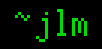## 2-Aug-2016

### Solving the quartic using “obvious” steps

Filed under: math — jlm @ 21:40

Looking over the derivations of solutions to quartics (fourth-degree polynomials), I was a little disturbed at how, except for Descartes’s, the derivations all have steps where “magic” occurs, by which I mean that a step does something mathematically valid but very unintuitive, which just happens to make stuff later on work out just right.

So I wondered whether one could take an approach like Ferrari’s, but do it without his “magic”. The basic idea to Ferrari’s approach is to convert the depressed* quartic equation f(x) = x⁴ + αx² + βx + γ = 0 into (x²+p)² = m(x+q)², because taking the square root of both sides produces the quadratic equation x²+p = ±(x+q)√m.

(x²+p)² = m(x+q)² expands into x⁴ + 2px² + p² = m(x²+2xq+q²), which is x⁴ + (2p−m)x² − 2mqx + p² − mq² = 0. We can name that polynomial g(x). From above, we know that the roots of g are the same as the roots of x² ± (x+q)√m + p, and the quadratic formula easily gives us those roots in terms of m, p, & q.

g ≡ f iff α = 2p−m and β = −2mq and γ = p²−mq². Thus our task is to get values for p, q, & m which satisfy these three equations.
m = −β/2q and p = ½(α+m), so p = ½(α − β/2q).
γ = p² − q²m = p² + q²β/2q = p² + ½βq = [½(α − β/2q)]² + ½βq = ¼[α² − αβ/q + β ²/4q² + 2βq].
4γq² = α²q² − αβq + ¼β ² + 2βq³.
2βq³ + (α² − 4γ)q² − αβq + ¼β ² = 0, which is just a cubic in q, which is something we’re presumed to know how to solve. Using q we get m and p from m=−β/2q and p=½(α+m).

With these values of m, p, & q, f ≡ g. So the roots of g(x) from above are the roots of f(x), et voilà, we’re done, no magic needed.

*A depressed nth degree polynomial is one in which the (n−1)th term has a coefficient of zero, so a depressed quartic is a quartic equation with no cubic term. For any nth degree polynomial F(x) = xn + an−1xn−1 + an−2xn−2 + …, there is a depressed nth degree polynomial f(x) = xn + bn−2xn−2 + … such that F(x+s) = f(x), because the coefficient of the (n−1)th term of F(x+s) is ns+an−1, which can always be made zero by a suitable choice of s.